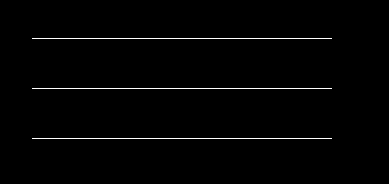# Draw a line in C++ graphics

graphics.h library is used to include and facilitate graphical operations in program. graphics.h functions can be used to draw different shapes, display text in different fonts, change colors and many more. Using functions of graphics.h you can make graphics programs, animations, projects and games. You can draw circles, lines, rectangles, bars and many other geometrical figures. You can change their colors using the available functions and fill them.

Examples:

```For line 1, Input : x1 = 150, y1 = 150, x2 = 450, y2 = 150
For line 2, Input : x1 = 150, y1 = 200, x2 = 450, y2 = 200
For line 2, Input : x1 = 150, y1 = 250, x2 = 450, y2 = 250
Output :```

## Recommended: Please try your approach on {IDE} first, before moving on to the solution.

Explanation :The header file graphics.h contains line() function which is described below :

Declaration : void line(int x1, int y1, int x2, int y2);

line function is used to draw a line from a point(x1,y1) to point(x2,y2) i.e. (x1,y1) and (x2,y2) are end points of the line.The code given below draws a line.

 `// C++ Implementation for drawing line ` `#include ` ` `  `// driver code ` `int` `main() ` `{ ` `    ``// gm is Graphics mode which is a computer display ` `    ``// mode that generates image using pixels. ` `    ``// DETECT is a macro defined in "graphics.h" header file ` `    ``int` `gd = DETECT, gm; ` ` `  `    ``// initgraph initializes the graphics system ` `    ``// by loading a graphics driver from disk ` `    ``initgraph(&gd, &gm, ``""``); ` ` `  `    ``// line for x1, y1, x2, y2 ` `    ``line(150, 150, 450, 150); ` ` `  `    ``// line for x1, y1, x2, y2 ` `    ``line(150, 200, 450, 200); ` ` `  `    ``// line for x1, y1, x2, y2 ` `    ``line(150, 250, 450, 250); ` ` `  `    ``getch(); ` ` `  `    ``// closegraph function closes the graphics ` `    ``// mode and deallocates all memory allocated ` `    ``// by graphics system . ` `    ``closegraph(); ` `} `

Output:

``````

My Personal Notes arrow_drop_upCheck out this Author's contributed articles.

If you like GeeksforGeeks and would like to contribute, you can also write an article using contribute.geeksforgeeks.org or mail your article to contribute@geeksforgeeks.org. See your article appearing on the GeeksforGeeks main page and help other Geeks.

Please Improve this article if you find anything incorrect by clicking on the "Improve Article" button below.

Article Tags :
Practice Tags :

2

Please write to us at contribute@geeksforgeeks.org to report any issue with the above content.